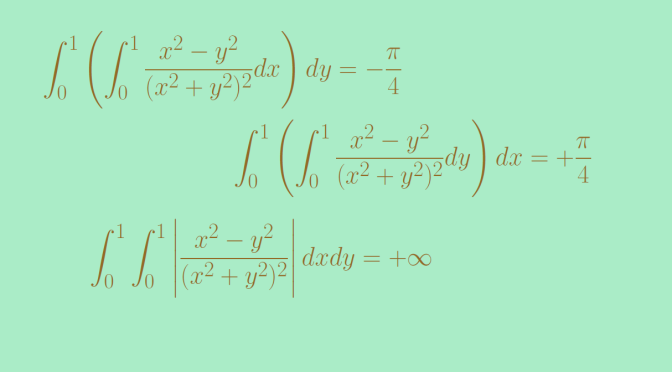Counterexamples around Fubini’s theorem

We present here some counterexamples around the Fubini theorem.

We recall Fubini’s theorem for integrable functions:
let $$X$$ and $$Y$$ be $$\sigma$$-finite measure spaces and suppose that $$X \times Y$$ is given the product measure. Let $$f$$ be a measurable function for the product measure. Then if $$f$$ is $$X \times Y$$ integrable, which means that $$\displaystyle \int_{X \times Y} \vert f(x,y) \vert d(x,y) < \infty$$, we have $\int_X \left( \int_Y f(x,y) dy \right) dx = \int_Y \left( \int_X f(x,y) dx \right) dy = \int_{X \times Y} f(x,y) d(x,y)$ Let's see what happens when some hypothesis of Fubini's theorem are not fulfilled.

The case of a measure that is not $$\sigma$$-finite

We take the set $$I=[0,1]$$ equipped on one side with the Borel set and Lebesgue measure $$\lambda$$ for $$X$$ and on the other side the same set $$I=[0,1]$$ equipped with the power set and the counting measure $$m$$ for $$Y$$.

The diagonal set $$\Delta=\{(x,x) \ : \ x \in [0,1] \}$$ is a closed subset of $$I^2$$. Hence $\Delta \in \mathcal{B}(I^2) = \mathcal{B}(I) \times \mathcal{B}(I) \subset \mathcal{B}(I) \times \mathcal{P}(I).$

Therefore, the characteristic map $$\mathbf{1}_{\Delta}$$ is measurable for the measure induced on $$X \times Y$$. However, we have on one side
\begin{align*}
\int_I \left( \int_I \mathbf{1}_{\Delta}(x,y) dm(y) \right) d\lambda(x) &= \int_I \left( \int_I \mathbf{1}_{\{x\}}(y) dm(y) \right) d\lambda(x)\\
&= \int_I m(\{x\}) d\lambda(x) = \lambda(I)=1
\end{align*}
and on the other side
\begin{align*}
\int_I \left( \int_I \mathbf{1}_{\Delta}(x,y) d\lambda(x) \right) dm(y) &= \int_I \left( \int_I \mathbf{1}_{\{y\}}(x) d\lambda(x) \right) dm(y)\\
&= \int_I \lambda(\{y\}) dm(y) = \int_I 0 \ dm(y)=0
\end{align*}

Those two integrals are different. This is not contradicting Fubini’s theorem as the counting measure on $$I=[0,1]$$ is not $$\sigma$$-finite. Indeed, a countable union of finite measure subsets for the counting measure is at most countable while $$I$$ has the cardinality of the continuum.

The case of a non-integrable function for the product measure

Let’s consider for $$X$$ and $$Y$$ the segment $$I=[0,1]$$ equipped with the Borel set $$\mathcal{B}(I)$$ and the Lebesgue measure. The function $f(x,y)=\frac{x^2-y^2}{(x^2+y^2)^2}.$ $$f$$ is continuous on $$I \times I$$ except at the origin, hence is measurable.

We have $\int_0^1 \frac{x^2-y^2}{(x^2+y^2)^2} dy = \left[ \frac{y}{x^2+y^2} \right]_{y=0}^1=\frac{1}{1+x^2}$ and $\int_0^1 \left(\int_0^1 \frac{x^2-y^2}{(x^2+y^2)^2} dy \right) dx = \int_0^1 \frac{1}{1+x^2} dx = \frac{\pi}{4}$ Proceeding to the integral computation in the opposite order we get $\int_0^1 \left(\int_0^1 \frac{x^2-y^2}{(x^2+y^2)^2} dx \right) dy = – \frac{\pi}{4}.$

Let’s have a look to $$\displaystyle \int_{I \times I} \vert f(x,y) \vert d(x,y)$$. We have
\begin{align*}
\int_{I \times I} \left\vert \frac{x^2-y^2}{(x^2+y^2)^2} \right\vert d(x,y) &\ge \int_0^1 \left(\int_0^ x\frac{x^2-y^2}{(x^2+y^2)^2} dy \right) dx\\
&=\int_0^1 \left[ \frac{y}{x^2+y^2} \right]_{y=0}^x dx\\
&= \int_0^1 \frac{1}{2x} dx = + \infty
\end{align*} avoiding any contradiction with Fubini’s theorem.